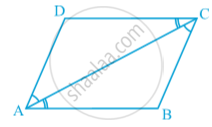# Properties of a Parallelogram - Theorem : a Diagonal of a Parallelogram Divides It into Two Congruent Triangles

#### theorem

Theorem: A diagonal of a parallelogram divides it into two congruent triangles.
Proof :  Let ABCD be a parallelogram and AC be a diagonal in following fig.Observe that the diagonal AC divides parallelogram ABCD into two triangles, namely, ∆ ABC and ∆ CDA. We need to prove that these triangles are congruent.
In ∆ ABC and ∆ CDA, note that BC || AD and AC is a transversal.
So, ∠ BCA = ∠ DAC (Pair of alternate angles)
Also, AB || DC and AC is a transversal.
So, ∠ BAC = ∠ DCA (Pair of alternate angles)
and AC = CA (Common)
So, ∆ ABC ≅ ∆ CDA (ASA rule)
or, diagonal AC divides parallelogram ABCD into two congruent triangles ABC and CDA.
Now, measure the opposite sides of parallelogram ABCD.
You will find that AB = DC and AD = BC.

If you would like to contribute notes or other learning material, please submit them using the button below.

### Shaalaa.com

Theorem - Diagonal divides parallelogram into two congruent triangles [00:07:28]
S
0%